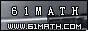Today is . WELCOME: htq777/44656  Home | Reg Login | All user | setHomePage | BookmarkHome | Math Test Online | Addition | Subtraction | Multiplication | Division | Time | Worksheet(JPG/PDF) | CAI | Flash Games |Recommendation articleArticle searchHome--maths--First Grade--Subtract Objects II

Subtract Objects II

Subtraction

Subtraction is removing some objects from a group. The meaning of 5-3=2 is "Three objects are taken away from a group of five objects and two objects remain".

Subtraction Facts

 Zero 0-0=0 One 1-0=1 1-1=0 Two 2-0=2 2-1=1 2-2=0 Three 3-0=3 3-1=2 3-2=1 3-3=0 Four 4-0=4 4-1=3 4-2=2 4-3=1 4-4=0 Five 5-0=5 5-1=4 5-2=3 5-3=2 5-4=1 5-5=0 Six 6-0=6 6-1=5 6-2=4 6-3=3 6-4=2 6-5=1 6-5=1 Seven 7-0=7 7-1=6 7-2=5 7-3=4 7-4=3 7-5=2 7-6=1 7-7=0 Eight 8-0=8 8-1=7 8-2=6 8-3=5 8-4=4 8-5=3 8-6=2 8-7=1 8-8=0 Nine 9-0=9 9-1=8 9-2=7 9-3=6 9-4=5 9-5=4 9-6=3 9-7=2 9-8=1 9-9=0 Ten 10-0=10 10-1=9 10-2=8 10-3=7 10-4=6 10-5=5 10-6=4 10-7=3 10-8=2 10-9=1 10-10=0

An example of subtraction. 8 - 5 = 3

 1 2 3 4 5 6 7 8 - 1 2 3 4 5 = 1 2 3

## Subtract the Number of Boxes.

-
=

2006-11-28 19:15:29
Viewed 1663 times CloseGreatmathsites